Introduction To Calculus

# Graphing Rational Functions

 1 Introduction 2 How to Find Asymptotes of a Rational Function 3 Graphing Rational Functions 4 Summary 5 About Cuemath 6 FAQs 7 External references

30 September 2020

## Introduction

If f(x) represents a rational expression then y = f(x) is a rational function. For graphing rational functions, we have to first find out the values for which the rational expression is undefined. A rational function is undefined for any values which make the denominator zero.

Let us start by graphing rational functions which are simple. Once you learn this we will be coming up with complex ones also.

First, let us know what a rational function is.

A rational function is a ratio of two polynomials:-

\begin{align}\frac{x^2+2}{x+3}\end{align}

It is a rational function as it can be expressed in a ratio form.

So A rational function is a ratio of two polynomials P(x) and Q(x):-

$F(x)=\frac{p(x)}{q(x)}$

Where Q(x)is not equal to 0

If you ever want to read it again as many times as you want, here is a downloadable PDF to explore more.

## How to Find Asymptotes of a Rational Function

To start with, let us get familiar with some terms involved in the rational graph.

What do you mean by asymptotes while graphing rational functions?

Rational function asymptotes

### Asymptote

It is a line that a curve approaches as it tends infinity.

Rational function asymptotes are of three types:- Vertical asymptote, Horizontal asymptote, and oblique.

### Vertical Asymptote

Vertical asymptote is a vertical line which corresponds to the zeros of the denominator of a rational function. It is a vertical straight line toward

Which a function approaches but never reaches or touches.

They correspond to the undefined locations of a rational function, or in an easy language set the denominator to zero and solve for x.

The below graph shows a vertical asymptote as x approaches some constant value c the curve goes towards infinity.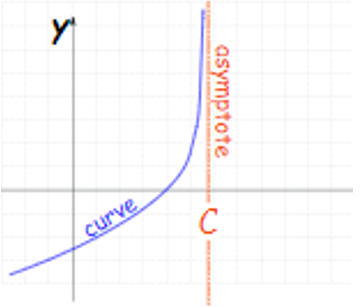### Horizontal Asymptote

Horizontal asymptotes are horizontal lines that the rational function graph of the rational expression tends to.

How to find the horizontal asymptote of a rational function?

As x tends to infinity and the curve approaches some constant value.As the name suggests they are parallel to the x axis.

Below x goes to infinity and the curve approaches some constant value b.

To answer how to find the horizontal asymptote of a rational function, we compare the degree of the numerator with the denominator of the rational expression.

\begin{align} F(x)=\frac{ax^n+...}{bx^m+...}\end{align}

If n < m then horizontal asymptote is the x axis $$(y=0)$$

If n = m then horizontal asymptote is $$(y=\frac{a}{b})$$

If n > m then there is no horizontal asymptote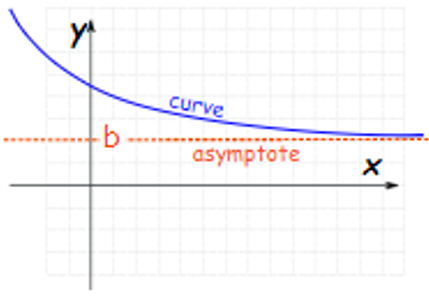### Oblique Asymptote

It is an oblique asymptote when

x goes to + infinity or – infinity then the curve goes towards a line

$Y = mx+b$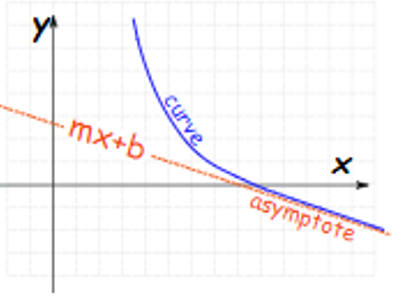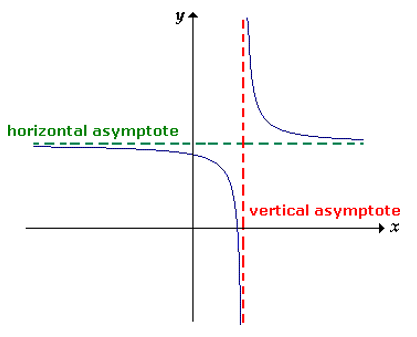Example of rational function asymptotes: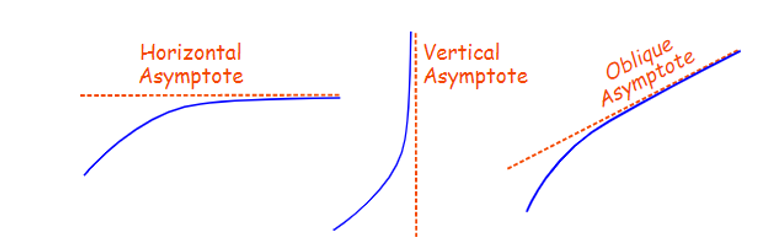## Graphing Rational Functions

To properly understand how to go about graphing rational functions, one must first know how to find asymptotes of a rational function, then the steps involved in potting the rational function graph. The simplest way to explain is by using actual rational expressions.

Now let us take an example:

 Example 1

How to graph a rational function:

$Y=\frac{2x+5}{x-1}$

Solution

Find out the vertical asymptote. Find the zeros of the denominator

So, upon observation, if x = 1 the denominator will be 0

We can not have x = 1 so we will have a vertical asymptote there. We can dash this on our graph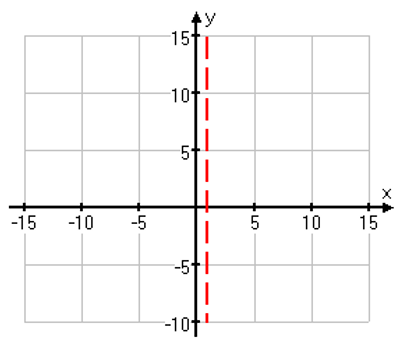Now we will find out the horizontal asymptote, the degree of the numerator and the denominator are equal the asymptote will be horizontal so

$Y=\frac{2}{1}$

We will dash this too.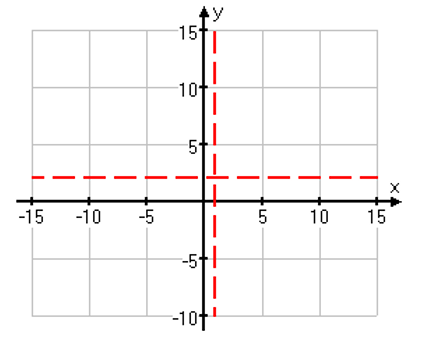Now we will find x and y intercepts

Putting the value of x = 0 in the rational function above we get

$y = \frac{5}{-1} = -5$

Putting y = 0

\begin{align}0& = \frac{(2x + 5)}{(x-1)}\\0 &= 2x + 5\\x &= \frac{-5}{2} = -2.5\end{align}

The intercepts are (0,-5) and (-2.5,0)

Now pick some more values of x and compute the corresponding values of y using the arational expression.

 x y -6 1 -1 -1.5 2 9 3 5.5 6 3.4

Let us plot these points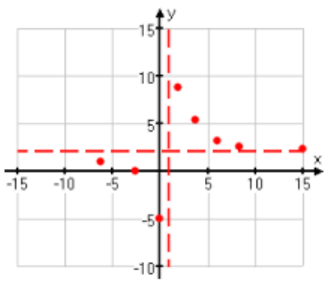Connecting the dots we get:-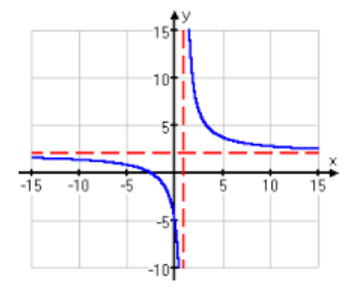How to graph a rational function?

Summarizing it in steps.

Step 1. Identify vertical asymptotes. (Find the zeros of the denominator)

Step 2. Check for horizontal asymptotes. (Compare the degrees of the numerator and the denominator)

Step 3. Plot points finding the intercepts. This will lead to complete graphing rational functions.

Here is a video that demonstrates Graphing Rational Functions

## Summary

We think that now you are familiar with the terms involved in graphing rational functions and the steps involved and you can develop a rational function graph. Try other such rational function graphs out. You can refer back to this blog and the procedure for graphing rational functions described until you fully grasp it. So keep doing such examples of a graphing rational function to keep you in practice.

To graph, a rational function is really easy. First, find out the intercepts if there are any, then find out the vertical asymptotes by setting the denominator equal to zero and the solving. Find the horizontal asymptote, if it exists, and then sketch the graph.

Written by Nitisha Sahai

Cuemath, a student-friendly mathematics platform, conducts regular Online Live Classes for academics and skill-development and their Mental Math App, on both iOS and Android, is a one-stop solution for kids to develop multiple skills. Understand the Cuemath Fee structure and sign up for a free trial.

## How to find the horizontal asymptote of a rational function?

Solution

To find the horizontal asymptote we, compare the degree of the numerator with the denominator.

$F(x)=\frac{ax^n+\dots}{bx^m+\dots}$

If n < m then horizontal asymptote is the x axis (y=0)

If n = m then horizontal asymptote is $$y=\frac{a}{b}$$

If n > m then there is no horizontal asymptote